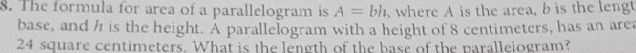### ¿Todavía tienes preguntas de matemáticas?

Pregunte a nuestros tutores expertos
Algebra
Pregunta8. The formula for area of a parallelogram is $$A =$$ bh. where $$A$$ is the area, $$b$$ is the lengt base, and $$h$$ is the height. A parallelogram with a height of $$8$$ centimeters, has an are.

$$24$$ square centimeters. What is the length of the basc of the naralleiogram?